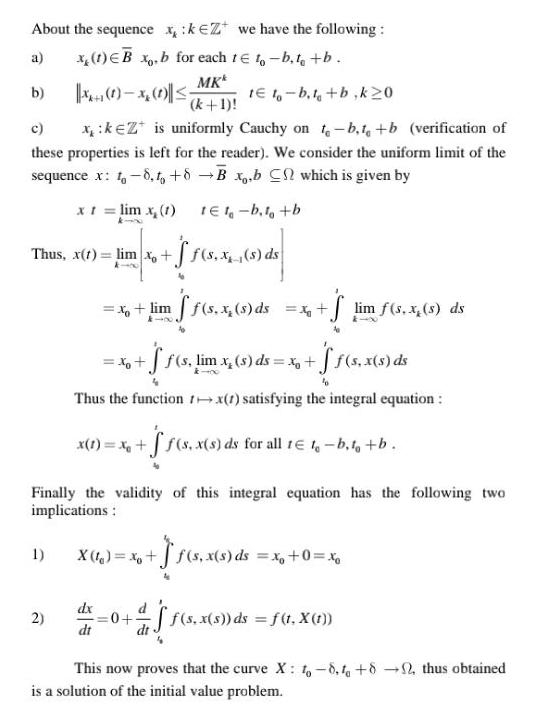Question:

# About the sequence x keZ we have the following a x 1 EB xo b

Last updated: 9/18/2023About the sequence x keZ we have the following a x 1 EB xo b for each te to b to b MKk b x x te t b t b k20 k 1 c xk Z is uniformly Cauchy on t b t b verification of these properties is left for the reader We consider the uniform limit of the sequence x t 8 to 8 B x b C which is given by x t lim x 1 1E1 b t b Thus x 1 lim x f s x s ds Finally the validity of this integral equation has the following two implications 1 x lim ff s x s ds x S lim f s x s ds x f f s lim x s ds x f f s x s ds k 400 Thus the function tx 1 satisfying the integral equation x 1 x f s x s ds for all t 1 b t b 2 X 1 x dx dt s x s ds s ds x 0 xo 0 as ff s x s ds f t X 1 This now proves that the curve X 1 8 to 82 thus obtained is a solution of the initial value problem